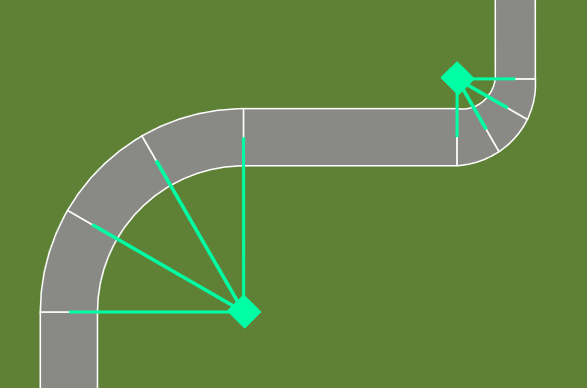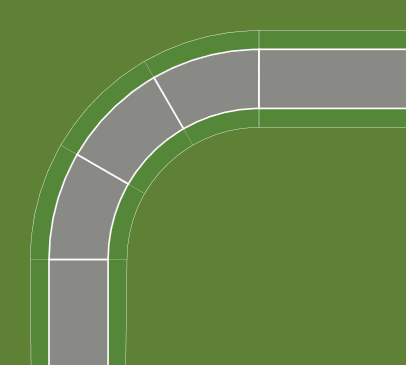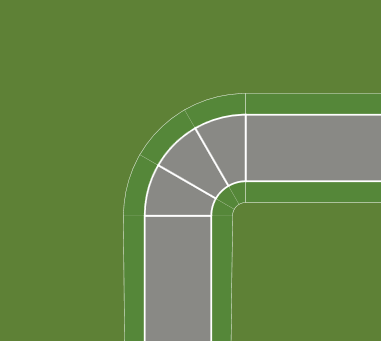# Track Corner Geometry

The two values in a track section that describe a corner in an F1GP track are the curvature and the length.

The curvature value defines how tight a corner is.

The allowed curvature value for a corner goes from -32,768 to 32,768, where a positive value indicates a right-hand corner, and a negative value indicates a left-hand turn. A value of 0 thus means that the section is a straight.

The curvature value is converted to indicate the length of the radius of an imagined circle on the inside of the corner, like this:In these two examples, the first corner has a smaller curvature value for the individual corner sections, and is therefore not as tight as the following corner, which has a larger curvature value.

The radius for an imagined circle on the inside of the corner is calculated according to the following formula:

1 / (curvature * 2 * 𝜋 / 65536)

1 10,430.378350470453
256 40.743665431525208
512 20.371832715762604
1024 10.185916357881302
2048 5.0929581789406511
2731 3.8192524168694444
4096 2.5464790894703255
8192 1.2732395447351628
16,384 0.63661977236758138
32,768 0.31830988618379069

So as you can see, the larger the curvature value, the smaller the radius.

### Example: 90° corners

To create a 90° corner, the curvature multiplied by the length must be approximately 16,384.

In the first example, we have a 90° corner with three sections.In this case, the curvature value for each section is 910, and the length of each section is 6.

This gives us the following calculation: 910 x 6 x 3 = 16,380.

In the next example, we have another 90° corner with three sections, but the corner is noticably tighter.The curvature value for these sections is larger than the previous one, 2,730, but the length is lower, at 2 each.

This gives us the following calculation: 2,730 x 2 x 3 = 16,380.

So, as you can see, the total value for each corner is the same but by adjusting the curvature and length values differently, the corners are tighter or wider.